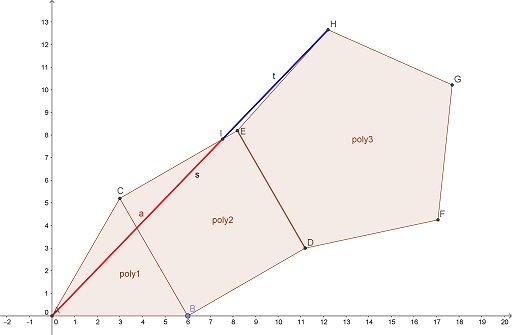# Golden Ratio in a Chain of Polygons, So to Speak

### Source

The following is another invention of Elliot McGucken.

Consider three regular polygons with $3,\;$ $4,\;$ and $5\;$ sides wherein all the polygons have sides of equal length throughout, as illustrated below.Then $\displaystyle\frac{AI}{IH}=\varphi,\;$ the Golden Ratio.

### Proof

I find it convenient to turn the diagram a little as to make the axis of symmetry more pronounced:Fighting the surge to apply the power of Analytic Geometry, let's have a closer look at what has to be shown. Introduce new notations as belowWhat needs to be shown is $\displaystyle\frac{a}{b}=\varphi.\;$ However, from triangles $AIK\;$ and $AHQ,\;$ $\displaystyle\frac{a+b}{a}=\frac{h_b}{h_a}=\varphi\;$ as the classical ratio of a diagonal and a side in a regular pentagon.

It follows that $\displaystyle\frac{a}{b}=\frac{1}{\varphi-1}=\varphi.$

### Remark

It is interesting to observe that the presence of a triangle and a square, however visually and intellectually appealing, is much less relevant to the appearance of the Golden Ratio than that of the pentagon. Indeed, for any position of $A\;$ on the axis of symmetry of the pentagon, the ratio will be the same, provided point $I\;$ on $AH\;$ is at the same distance from the axis as the vertex $E:$To illustrate that point and separate of what's important from what is not, Tran Quang Hung came up with the following construction: in a chain of a regular hexagon, a square, and a regular pentagon,$AB=BC,\;$ and the reason is immediately clear from the following diagram by the same reasoning as above.Tran Quang Hung has also observed that the Golden Ratio is cut off by a perpendicular bisector in a combination of a square and a regular pentagon:The assertion was christened "Bishop's theorem" by Gae Spes. It is clear that a rectangle can be used instead of a square.

### Golden Ratio